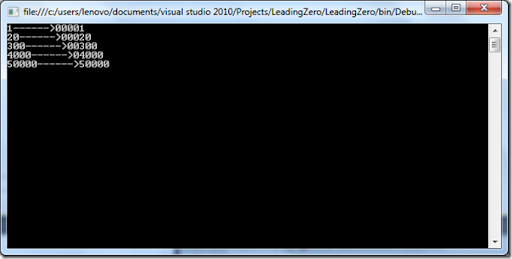Wednesday, April 4, 2012

Recently I was working with a project where I was in need to format a number in such a way which can apply leading zero for particular format. So after doing such R and D I have found a great way to apply this leading zero format.

I was having need that I need to pad number in 5 digit format. So following is a table in which format I need my leading zero format.

1-> 00001
20->00020
300->00300
4000->04000
50000->5000

So in the above example you can see that 1 will become 00001 and 20 will become 00200 format so on. So to display an integer value in decimal format I have applied interger.Tostring(String) method where I have passed “Dn” as the value of the format parameter, where n represents the minimum length of the string. So if we pass 5 it will have padding up to 5 digits.

So let’s create a simple console application and see how its works. Following is a code for that.

```using System;

{
class Program
{
static void Main(string[] args)
{
int a = 1;
int b = 20;
int c = 300;
int d = 4000;
int e = 50000;

Console.WriteLine(string.Format("{0}------>{1}",a,a.ToString("D5")));
Console.WriteLine(string.Format("{0}------>{1}", b, b.ToString("D5")));
Console.WriteLine(string.Format("{0}------>{1}", c, c.ToString("D5")));
Console.WriteLine(string.Format("{0}------>{1}", d, d.ToString("D5")));
Console.WriteLine(string.Format("{0}------>{1}", e, e.ToString("D5")));
}
}
}```

As you can see in the above code I have use string.Format function to display value of integer and after using integer value’s ToString method. Now Let’s run the console application and following is the output as expected.Here you can see the integer number are converted into the exact output that we requires. That’s it you can see it’s very easy. We have written code in nice clean way and without writing any extra code or loop. Hope you liked it. Stay tuned for more.. Till than happy programming.Share:

1.thanks
it's working

1.You are welcome